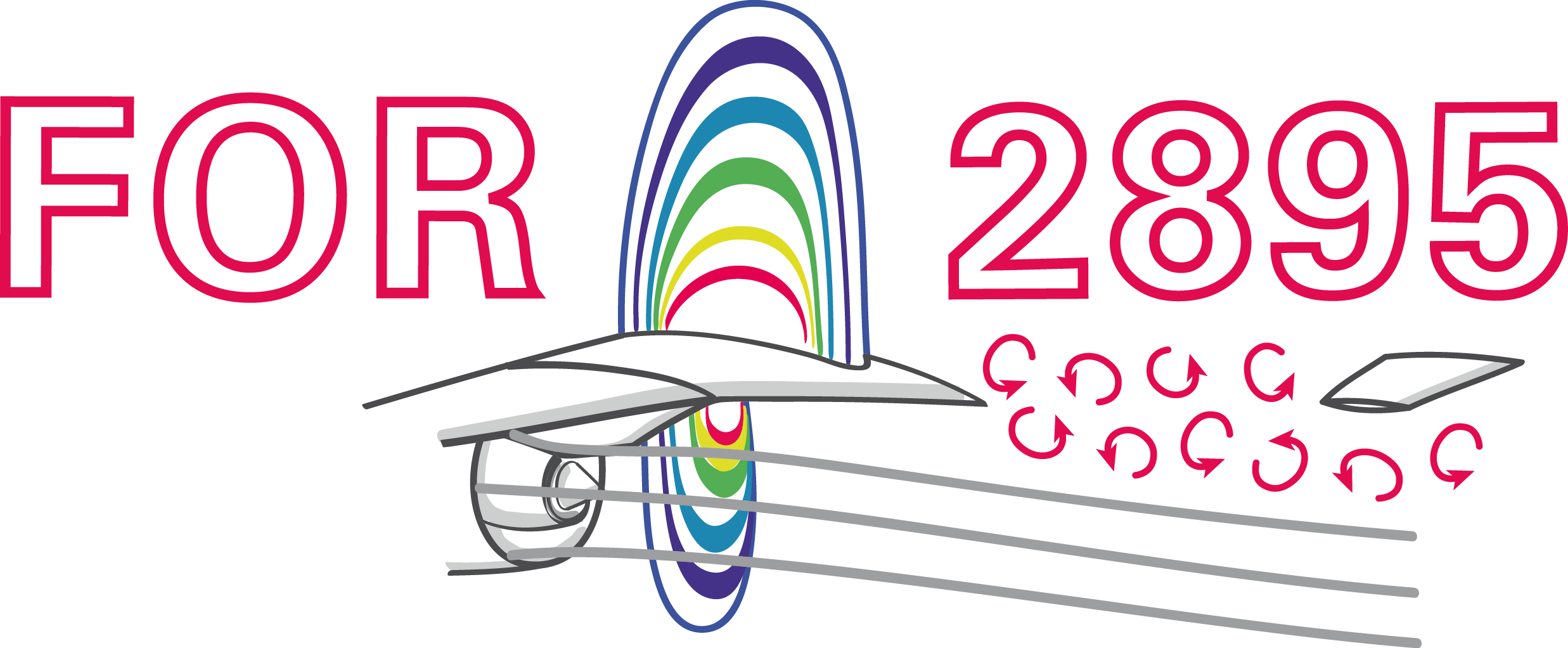### Setting up a RL problem from scratch with drlfoam

Andre Weiner, Tomislav Maric
TU Braunschweig, Institute of Fluid Mechanics

## Outline

1. closed-loop active flow control
2. (deep) reinforcement learning
3. setting up a flow control problem
• OF simulation
• OF boundary condition
• drlFoam environment
4. running a training
5. what's next?

## Closed-loop active flow control

Goals of flow control:

• drag reduction
• process intensification
• noise reduction
• ...

Categories of flow control:

1. passive: geometry, fluid properties, ...
2. active: blowing/suction, heating/cooling, ...

active: energy input vs. efficiency gain

Categories of active flow control:

1. open-loop: actuation predefined
2. closed-loop: actuation based on sensor input

How to find the control law?

Closed-loop flow control with variable Reynolds number; source: F. Gabriel 2021.

Why CFD-based DRL?

• save virtual environment
• prior optimization, e.g., sensor placement

## deep reinforcement learningCreate an intelligent agent that learns to map states to actions such that expected returns are maximized.

$s_t$ - state $s$ at time $t$ (observation)

• velocity probes
• surface pressure probes
• temperature probes
• ...

$s$ should be low-dimensional

$a_t$ - action $a$ at time $t$

• angular velocity
• mass flux
• heat flux
• ...

$a$ should be low-dimensional

reward and reward function

$r=-c_d - 0.1 |c_l|$

$r_t$ - reward at time $t$

$c_d$, $c_l$ - drag and lift coefficient

experience tuple

$$\left\{ s_t, a_t, r_{t+1}, s_{t+1}\right\}$$

trajectory

$\left\{s_0, a_0, r_1, s_1\right\}$
$\left\{s_1, a_1, r_2, s_3\right\}$
$\left\{ ...\right\}$

Long-term consequences:

$$G_t = \sum\limits_{l=0}^{N_t-t} \gamma^l r_{t+l}$$

• $t$ - control time step
• $G_t$ - discounted return
• $\gamma$ - discount factor, typically $\gamma=0.99$
• $N_t$ - number of control steps

What to expect in a given state?

$$L_V = \frac{1}{N_\tau N_t} \sum\limits_{\tau = 1}^{N_\tau}\sum\limits_{t = 1}^{N_t} \left( V(s_t^\tau) - G_t^\tau \right)^2$$

• $\tau$ - trajectory (single simulation)
• $s_t$ - state/observation (pressure)
• $V$ - parametrized value function
• clipping not included

Was the selected action a good one?

$$\delta_t = R_t + \gamma V(s_{t+1}) - V(s_t)$$ $$A_t^{GAE} = \sum\limits_{l=0}^{N_t-t} (\gamma \lambda)^l \delta_{t+l}$$

• $\delta_t$ - one-step advantage estimate
• $A_t^{GAE}$ - generalized advantage estimate
• $\lambda$ - smoothing parameter

Making good actions more likely:

$$J_\pi = \frac{1}{N_\tau N_t} \sum\limits_{\tau = 1}^{N_\tau}\sum\limits_{t = 1}^{N_t} \left( \frac{\pi(a_t|s_t)}{\pi^{old}(a_t|s_t)} A^{GAE,\tau}_t\right)$$

• $\pi$ - current policy
• $\pi^{old}$ - old policy (previous episode)
• clipping and entropy not included
• $J_\pi$ is maximized

## setting up a flow control problem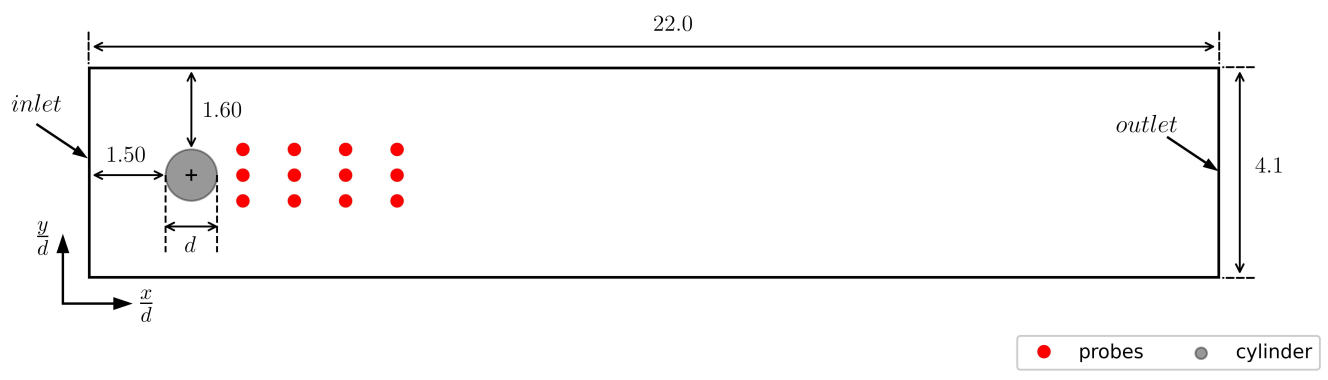Cylinder benchmark case; $Re=100$.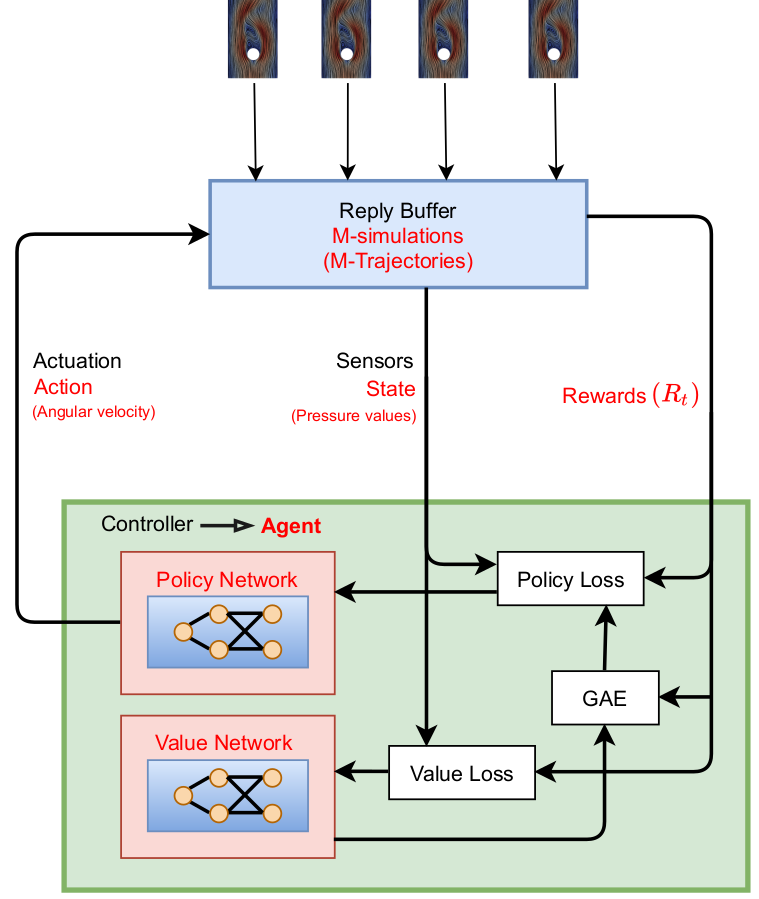Proximal policy optimization (PPO) workflow (GAE - generalized advantage estimate).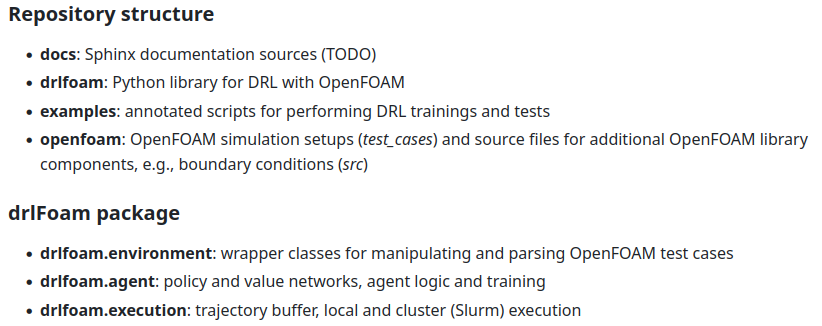github.com/OFDataCommittee/drlfoam

3 steps to solve a new control problem

1. OpenFOAM simulation
2. boundary condition (C++)
3. drlfoam Env (Python)

### 1. OF simulation

openfoam/test_cases/rotatingCylinder2D

inputs for control law


probes
{
type            probes;
libs            (sampling);
executeControl  runTime;
executeInterval 0.01;
writeControl    runTime;
writeInterval   0.01;
timeStart       4.0;
fields          (p);
probeLocations
(
(0.3 0.15 0.005)
...
);
}


quantities defining control objective


forces
{
type            forceCoeffs;
libs            (forces);
executeControl  runTime;
executeInterval 0.01;
writeControl    runTime;
writeInterval   0.01;
timeStart       4.0;
patches
(
cylinder
);
coefficients    (Cd Cl);
...
}


define how to run the simulation

• Allrun.pre
• Allrun
• Allclean

### 2. boundary condition

openfoam/src/agentRotatingWallVelocity

getting the state


if (timeToControl && update_omega_)
{
const volScalarField& p =
this->db().lookupObject<volScalarField>("p");
scalarField p_sample = probes_.sample(p);
...
}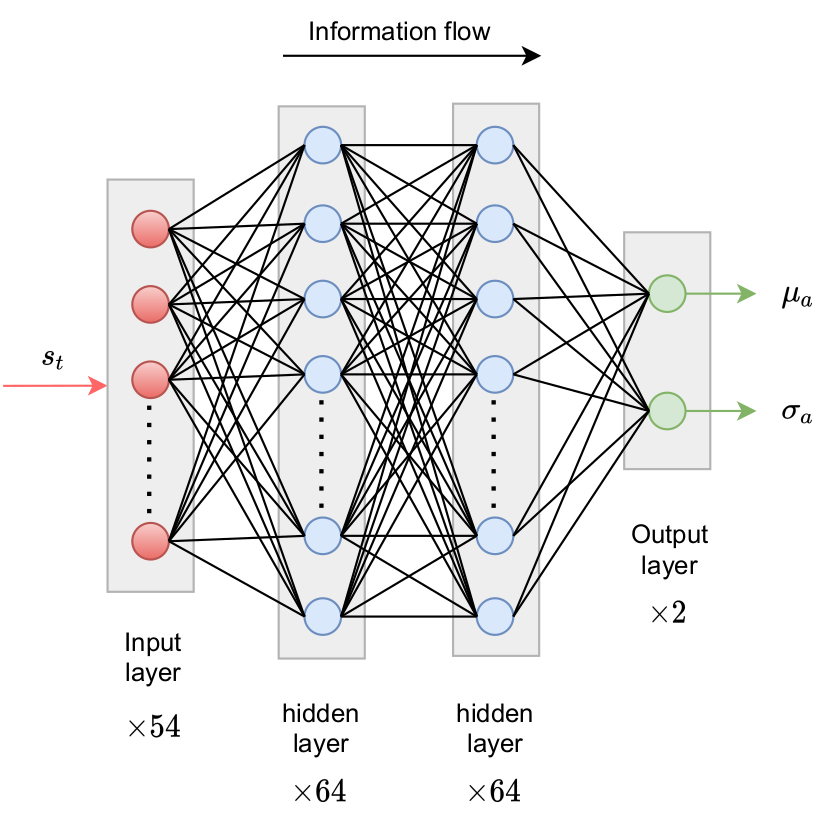Policy network predicts probability density function(s) for action(s).Comparison of Gauss and Beta distribution.

getting the next action I


torch::Tensor features = torch::from_blob(
p_sample.data(), {1, p_sample.size()},
torch::TensorOptions().dtype(torch::kFloat64)
);
std::vector<torch::jit::IValue> policyFeatures{features};
torch::Tensor dist_parameters = policy_.forward(policyFeatures).toTensor();
scalar alpha = dist_parameters.item<double>();
scalar beta = dist_parameters.item<double>();


getting the next action II


std::gamma_distribution<double> distribution_1(alpha, 1.0);
std::gamma_distribution<double> distribution_2(beta, 1.0);
scalar omega_pre_scale;
if (train_)
{
// sample from Beta distribution during training
double number_1 = distribution_1(gen_);
double number_2 = distribution_2(gen_);
omega_pre_scale = number_1 / (number_1 + number_2);
}
else
{
// use expected (mean) angular velocity
omega_pre_scale = alpha / (alpha + beta);
}
// rescale to actionspace
omega_ = (omega_pre_scale - 0.5) * 2 * abs_omega_max_;


using the boundary condition in 0.org/U


cylinder
{
type            agentRotatingWallVelocity;
origin          (0.2 0.2 0.0);
axis            (0 0 1);
policy          "policy.pt";
probesDict      "probes";
train           true;
seed            0;
absOmegaMax     5.0;
value           uniform (0 0 0);
}


### drlfoam Env

drlfoam/environment/rotating_cylinder.py

some convenience functions


def _parse_forces(path: str) -> DataFrame:
return forces

def _parse_probes(path: str, n_probes: int) -> DataFrame:
names = ["t"] + [f"p{i}" for i in range(n_probes)]
comment="#", delim_whitespace=True
)


derived class


class RotatingCylinder2D(Environment):
def __init__(self, r1: float = 3.0,
r2: float = 1.0, r3: float=0.1):
super(RotatingCylinder2D, self).__init__(
join(TESTCASE_PATH, "rotatingCylinder2D"),
"Allrun.pre", "Allrun", "Allclean", 2, 12, 1
)
...

def _reward(self, cd: pt.Tensor, cl: pt.Tensor) -> pt.Tensor:
return self._r1 - (self._r2 * cd + self._r3 * cl.abs())
...


## running a training

how to run a training

• go to examples
• modify run_example.py

DEFAULT_CONFIG = {
"rotatingCylinder2D" : {
"policy_dict" : {
"n_layers": 2,
"n_neurons": 64,
"activation": pt.nn.functional.relu
},
"value_dict" : {
"n_layers": 2,
"n_neurons": 64,
"activation": pt.nn.functional.relu
}
}
...


config = SlurmConfig(
n_nodes=1,
partition="queue-1",
time="03:00:00",
constraint="c5a.24xlarge",
modules=["openmpi/4.1.5"],
commands_pre=[
"source /fsx/OpenFOAM/OpenFOAM-v2206/etc/bashrc",\
"source /fsx/drlfoam_main/setup-env"
]
)
buffer = SlurmBuffer(training_path, ...)


#!/bin/bash
#SBATCH --job-name=train_cylinder
#SBATCH --output=%x_%j.out
#SBATCH --partition=queue-1
#SBATCH --constraint=c5a.24xlarge

source /fsx/OpenFOAM/OpenFOAM-v2206/etc/bashrc
source /fsx/drlfoam_main/setup-env
source /fsx/drlfoam_main/pydrl/bin/activate

python3 run_training.py -o first_training \
-i 100 -r 10 -b 10 -f 8 \
-e slurm &> log.first_training


evaluating a policy

• make a copy of base and clean up
• copy policy_trace_xxx.pt
to the new folder; name it policy.pt
• set train 0; in 0.org/U
• adjust timeStart ...; in system/
• execute Allrun.pre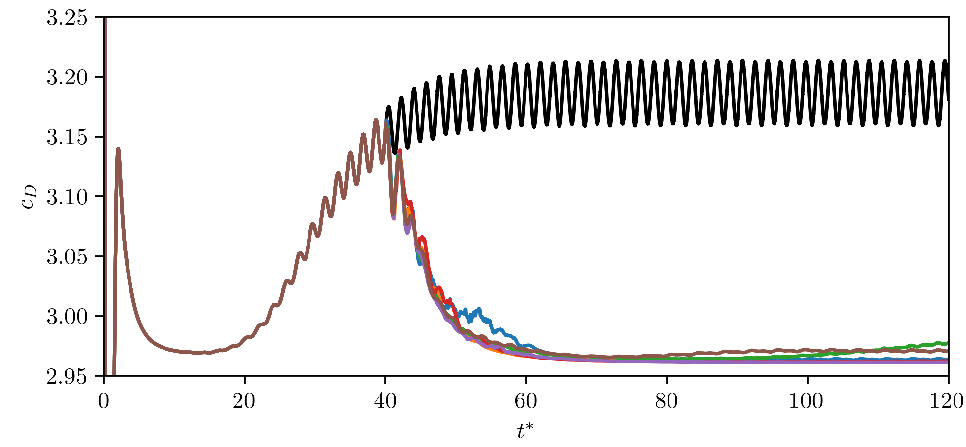Comparison of controlled and uncontrolled drag coefficient.

Evaluation of final policy.

## What's next?

more control problems

• turbulence modeling
• shape optimization
• linear solvers
• ...

$\rightarrow$ Advances in the application of reinforcement learning to flow control problems

Wed, 09:00-09:20, room 4H

data-driven modeling SIG meeting

Thu, 5:15-6:15 pm, room 4H

3rd OpenFOAM-ML hackathon (register)

Jul 24-26

thanks to

Darshan Thummar, Fabian Gabriel, Jan Erik Schulze, Tom Krogmann, Janis Geise

Ajay Navilarekal Rajgopal, Guilherme Lindner, Julian Bissantz, Morgan Kerhouant, Mosayeb Shams, Tomislav Marić

Neil Ashton

# THE END

### Thank you for you attention!